# ISEE Lower Level Quantitative : Identifying Place Value

## Example Questions

← Previous 1 3 4 5 6 7 8 9 70 71

### Example Question #1 : Understand Place Value For Three Digit Numbers: Ccss.Math.Content.2.Nbt.A.1

What digit is in the hundreds place?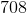Explanation:

The hundreds place is always the third number over from the right. In this case,is the third number over from the right.

### Example Question #1 : Number & Operations In Base Ten

What digit is in the tens place?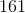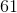Explanation:

The tens place is always the second number over from the right. In this case,is the second number over from the right.

### Example Question #3 : Understand Place Value For Three Digit Numbers: Ccss.Math.Content.2.Nbt.A.1

What digit is in the ones place?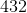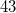Explanation:

The ones place is always the first number on the right. In this case,is the first number on the right.

### Example Question #4 : Understand Place Value For Three Digit Numbers: Ccss.Math.Content.2.Nbt.A.1

What digit in the hundreds place?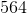Explanation:

The hundreds place is always the third number over from the right. In this case,is the third number over from the right.

### Example Question #1 : Understand Place Value For Three Digit Numbers: Ccss.Math.Content.2.Nbt.A.1

What digit is in the tens place?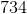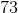Explanation:

The tens place is always the second number over from the right. In this case,is the second number over from the right.

### Example Question #6 : Understand Place Value For Three Digit Numbers: Ccss.Math.Content.2.Nbt.A.1

What digit is in the ones place?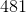Explanation:

The ones place is always the first number on the right. In this case,is the first number on the right.

### Example Question #2 : Number & Operations In Base Ten

What digit is in the hundreds place?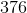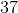Explanation:

The hundreds place is always the third number over from the right. In this case,is the third number over from the right.

### Example Question #1 : Understand Place Value For Three Digit Numbers: Ccss.Math.Content.2.Nbt.A.1

What digit is in the tens place?Explanation:

The tens place is always the second number over from the right. In this case,is the second number over from the right.

### Example Question #9 : Understand Place Value For Three Digit Numbers: Ccss.Math.Content.2.Nbt.A.1

What digit is in the ones place?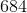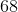Explanation:

The ones place is always the first number on the right. In this case,is the first number on the right.

### Example Question #10 : Understand Place Value For Three Digit Numbers: Ccss.Math.Content.2.Nbt.A.1

What digit is in the hundreds place?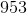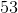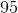The hundreds place is always the third number over from the right. In this case,is the third number over from the right.